# 2. 向量的表示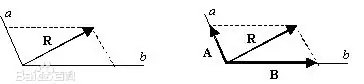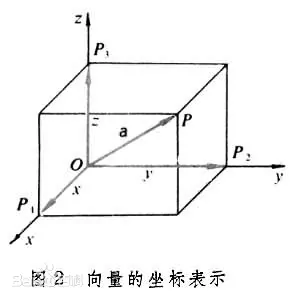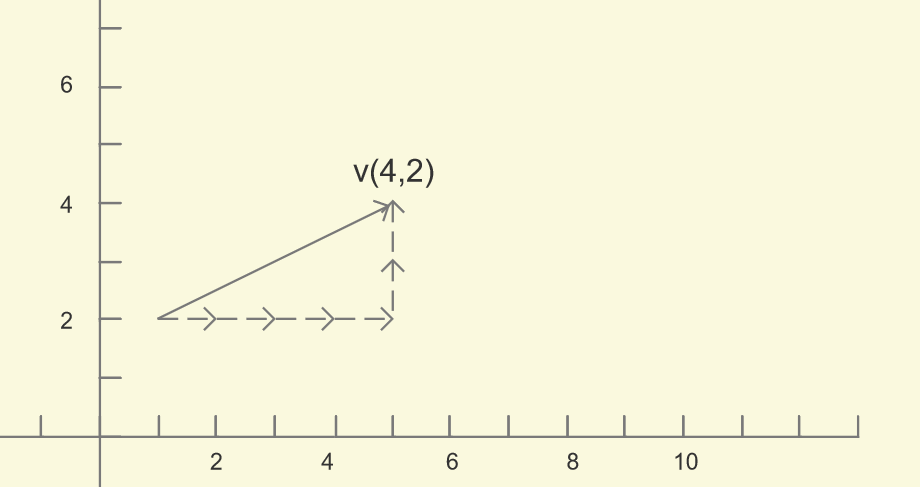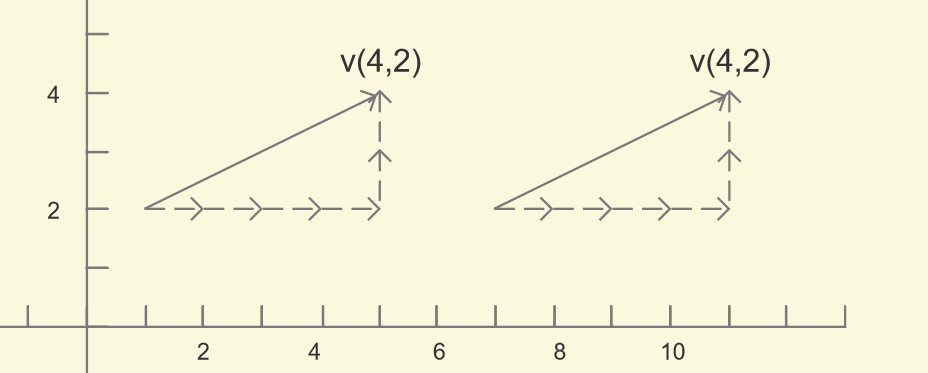# 3. 向量的求解

v(a, b) = point1 - point2 = v(point1.x - point2.x, point1.y - point2.y)

v(2, 2):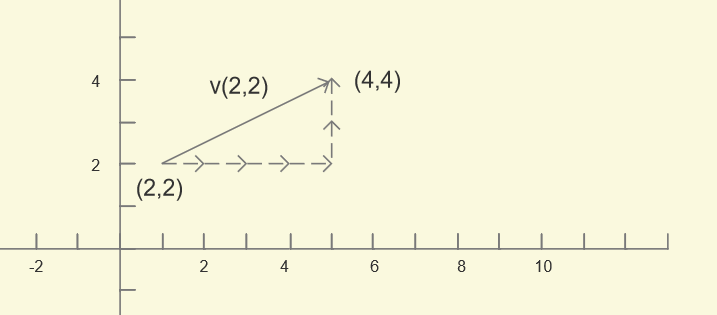v(-2. -2):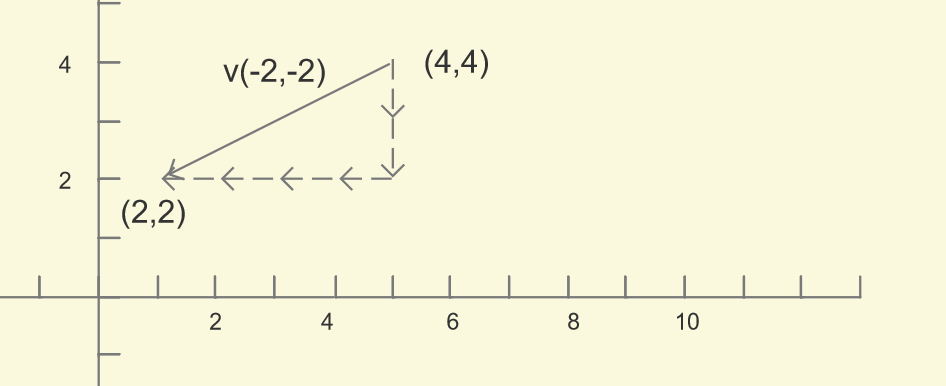# 4. 向量的四则运算

## 4.1 加法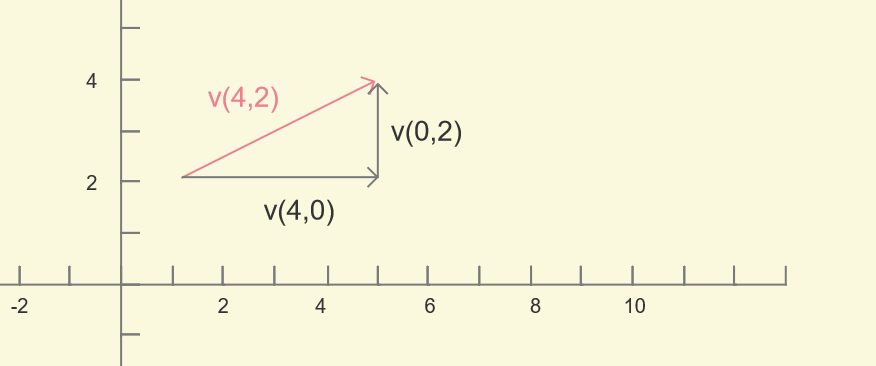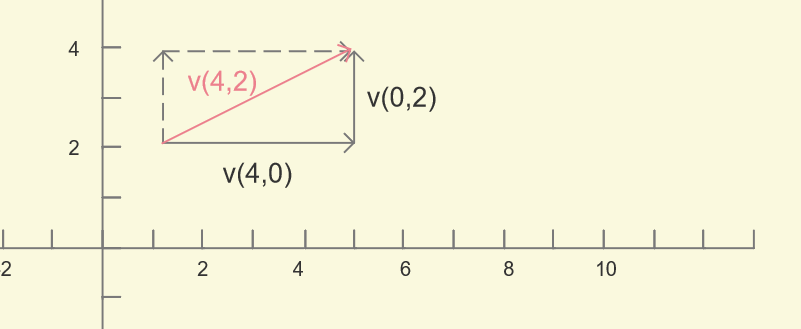/**
* */

public Vector add( Vector vector ) {
// 无视minsID
return new Vector( x + vector.x, y + vector.y, minsID-- );
}


## 4.2 减法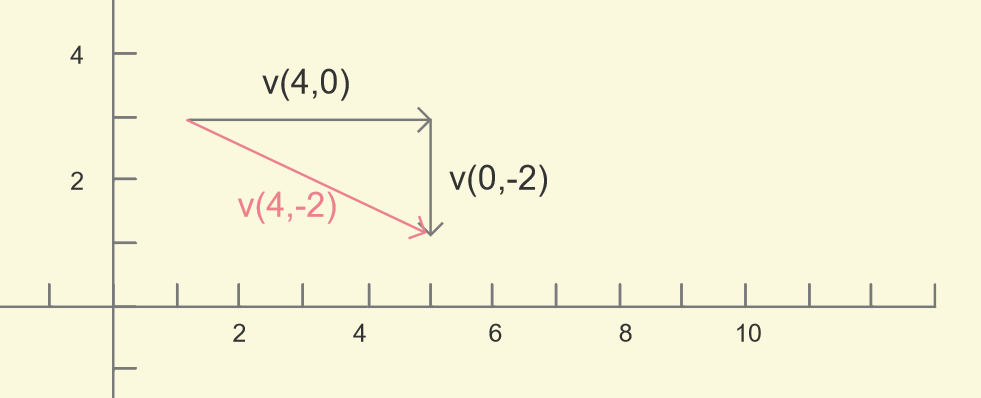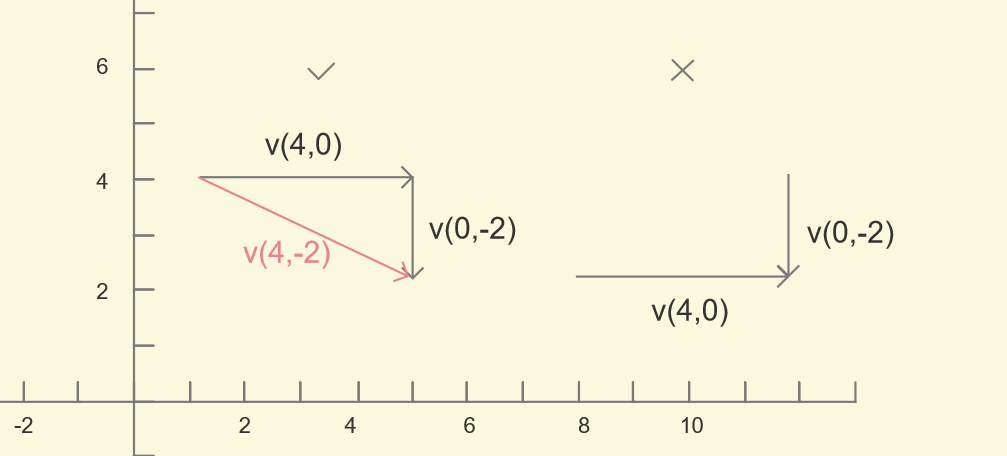/**
* vector subtract
* */

public Vector subtract( Vector vector ) {
return new Vector( x - vector.x, y - vector.y, minsID-- );
}


## 4.3 乘法和除法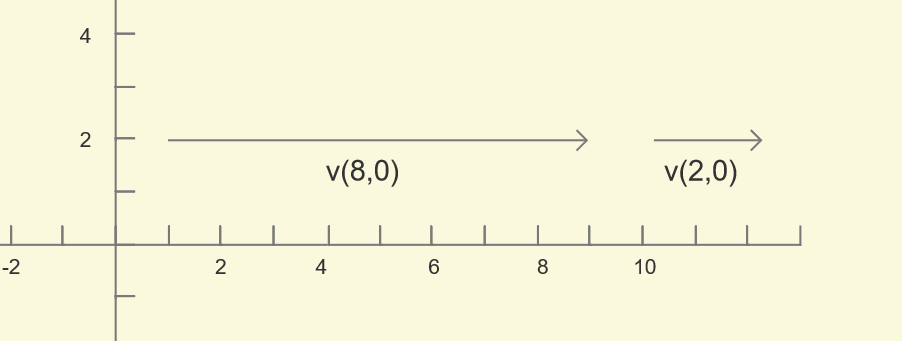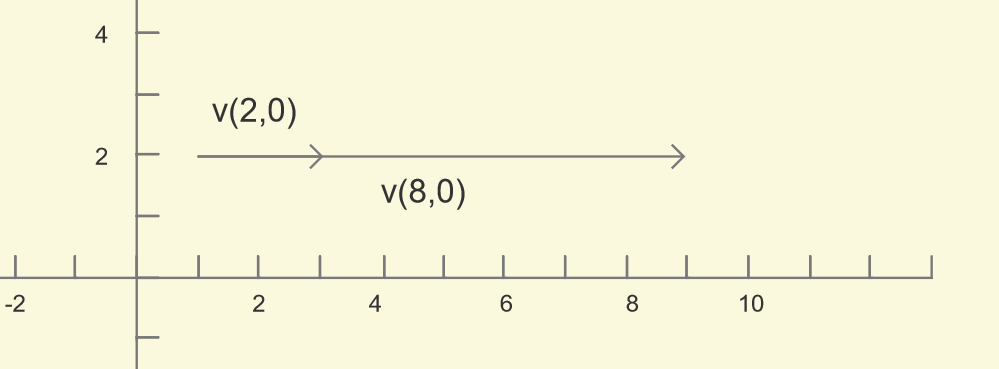/**
* vector multiplication
* */

public Vector multiply( float ratio ) {
return new Vector( ratio * x , ratio * y, minsID-- );
}

/**
* vector division
* */

public Vector division( float ratio ) {
return new Vector( x / ratio , y / ratio, minsID-- );
}


# 5. 点积和叉积

## 5.1 点积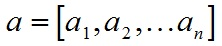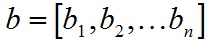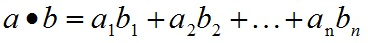/**
* dot multiplication, vector * vector
* */

public static float dot( Vector vector1, Vector vector2 ) {
return vector1.x * vector2.x + vector1.y * vector2.y;
}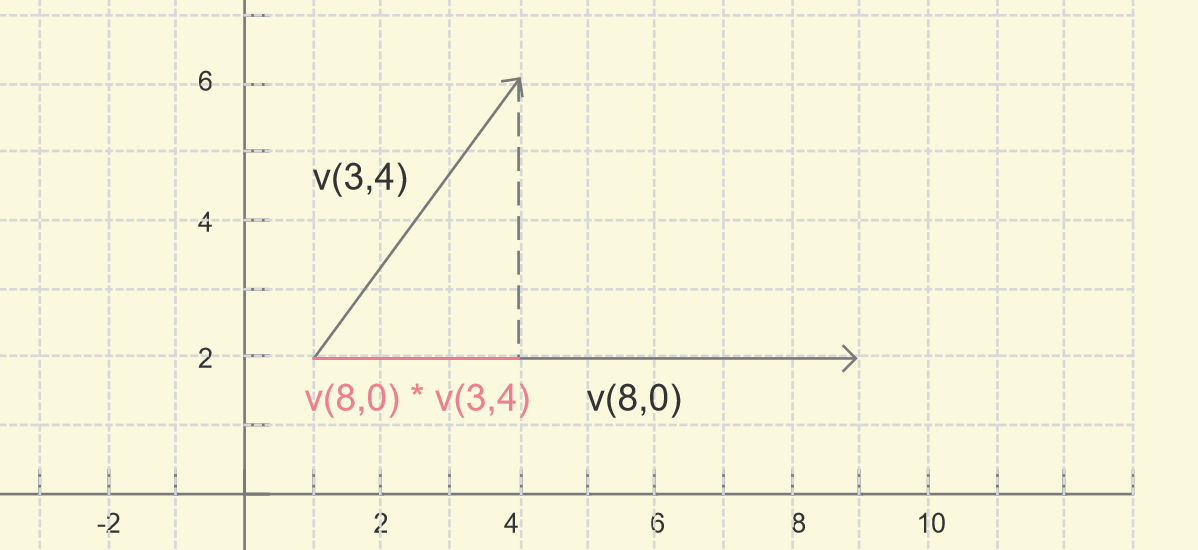## 5.2 叉积

/**
* cross multiplication,  vector x vector
* */

public static float cross( Vector vector1, Vector vector2 ) {
return vector1.x * vector2.y - vector1.y * vector2.x;
}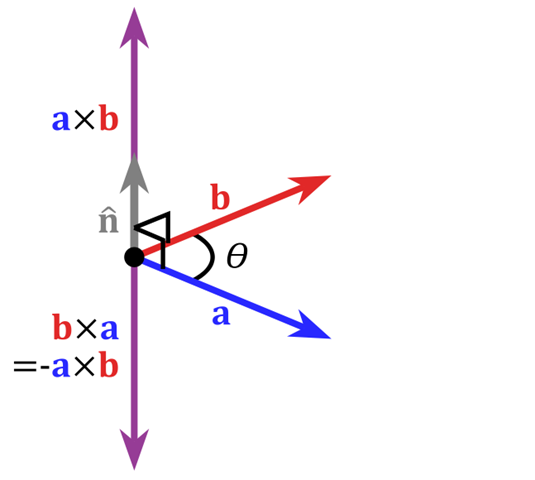# 6. 模的求解

/**
* vector's norm, but without radical
* */

return x * x + y * y;
}

/**
* vector's norm
* */

public float norm() {
return ( float ) Math.sqrt( normWithoutRadical() );
}


e(a, c) = v(a, b) / |v(a, b)|

# 7. 附录：代码

1. 代码：Vector类
2. 项目代码：Algorithm Repository

# 8. 免责声明

※ 本文之中如有错误和不准确的地方，欢迎大家指正哒~
※ 此项目仅用于学习交流，请不要用于任何形式的商用用途，谢谢呢；12-071万+
05-176493

07-043万+
05-23200
07-11201
11-061万+
09-0271万+
02-112086
09-091566
03-25200
08-256068
03-031919
09-217415
11-02910
02-196万+
04-129544
05-262万+

### “相关推荐”对你有帮助么？

•非常没帮助
•没帮助
•一般
•有帮助
•非常有帮助fengkeyleaf

¥2 ¥4 ¥6 ¥10 ¥20余额支付 (余额：-- )扫码支付获取中扫码支付点击重新获取扫码支付1.余额是钱包充值的虚拟货币，按照1:1的比例进行支付金额的抵扣。
2.余额无法直接购买下载，可以购买VIP、C币套餐、付费专栏及课程。余额充值